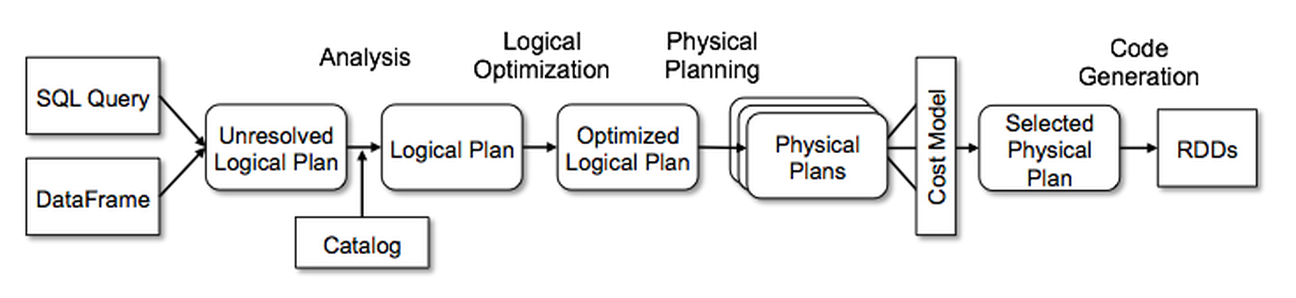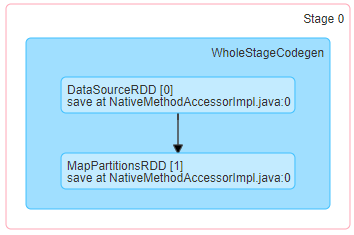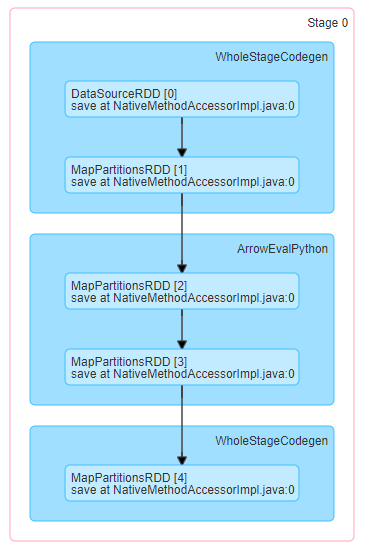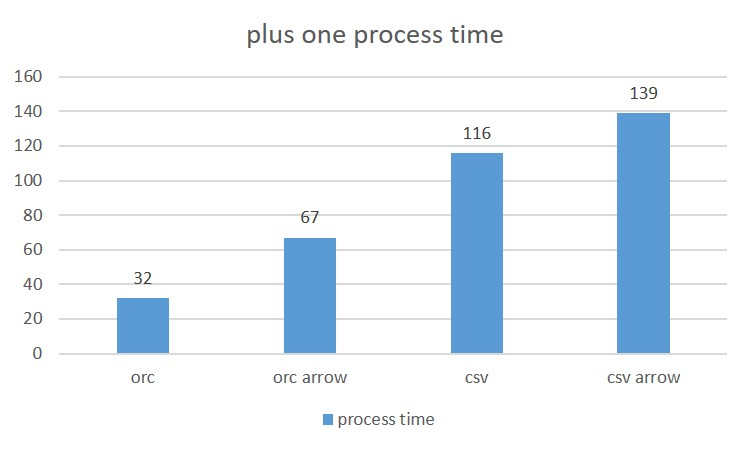# Chendi Xue

I am linux software engineer, currently working on Spark, Arrow, Kubernetes, Ceph, c/c++, and etc.

16 Apr 2019

Reference: Deep Dive into Spark Storage formats

### How spark handles sql requestFrom above article, we can see that a spark sql will go though Analysis, Optimizer, Physical Planning then using Code Generation to turn into RDD java codes. There is a greate amount of rules and ops inside above steps for different type of input data and different transition sql asks.

Below, I used a very simple example of plusing one to each line of a two column table to see what codes would be generated.

1. columnar data layout: Orc or Parquet
2. csv data
3. csv with Arrow

### Columnar Data Layout

Pyspark codes

``````from pyspark.sql import SparkSession
from pyspark.sql.functions import udf
from pyspark.sql.types import IntegerType

def plus_one_func(v):
return v + 1

spark = SparkSession.builder.appName("pyspark_plus_one_orc").getOrCreate()
df = df.withColumn('count', plus_one_func(df["count"]))
df.write.format("parquet").save("/HiBench/DataFrame/Output")
``````

After Spark CodeGen gist url, the task will be generated as below.

``````public Object generate(Object[] references) {
return new GeneratedIteratorForCodegenStage1(references);
}

final class GeneratedIteratorForCodegenStage1 extends org.apache.spark.sql.execution.BufferedRowIterator {
// define variables ...
public GeneratedIteratorForCodegenStage1(Object[] references){...}
public void init(int index, scala.collection.Iterator[] inputs){
result = new org.apache.spark.sql.catalyst.expressions.codegen.UnsafeRowWriter(2, 32);
}

protected void processNext() throws java.io.IOException {
// CodeGenStage1 is a BufferedRowIterator
int batchIdx = 0;
while ( columnarBatch != null ) {
// Notice:
// columnarBatch points to the next value of the inputsIterator, which is a scala.collection.Iterator
// One Iterator Row in this case is one column Batch, because we used Orc. Case is same if using Parquet.
int numRows = columnarBatch.numRows(); // numRows should be end row number of this columnarBatch.
int localEnd = numRows - batchIdx;
for (int rowIdx = 0; rowIdx < localEnd; rowIdx ++) {
rowIdx += batchIdx;
org.apache.spark.sql.vectorized.ColumnVector columeVector_0 = columnarBatch.column(0);
org.apache.spark.sql.vectorized.ColumnVector columeVector_1 = columnarBatch.column(1);
UTF8String  first_column = columeVector_0.getUTF8String(rowIdx_0);
int second_column = columeVector_1.getInt(rowIdx_0);
project_value_1 = second_column + 1;
// PLUS ONE is Processed by looping in per value in column!!!
}
// write to result
result.write(0, first_column);
result.write(1, project_value_1);

batchIdx = numRows;
batchscan_nextBatch_0(); // get next item in Iterator as columeBatch
}
}

private void batchscan_nextBatch_0() throws java.io.IOException {
org.apache.spark.sql.vectorized.ColumnarBatch columnarBatch = scala.collection.Iterator.next();
}
}
``````

There is only one code gen stage, Although this is pyspark script, since plus_one is simple, it handled as expression inside JVM.### Findings:

1. When using orc or parquet as input data, org.apache.spark.sql.vectorized.ColumnarBatch and ColumnVector will be used.
2. For plus_one operation, data was processed batch by batch then row by row inside one batch (CPU optimizable?).

### orc with arrow

ORC with Arrow Generated codes

When processing orc data with Arrow(pandas_udf), there will be two codegen stages. One is before ArrowEval, and one is after. So before ArrowEval stage codegen will treat data as columnarBatch, and each row inside columnVector batch will be wrote into sql.catalyst.expressions.codegen.UnsafeRowWriter one by one. After ArrowEval, input seems to be row based, (TODO: check inputadapter_input_0.next() for exact data layout)### CSV

CSV Generated codes

1. When using csv(row-based) data, input collection will be processed one row by one row(no batch).
2. plus one is processed inside JVM as expression, so only one codegen stage created here.

### CSV with Arrow

CSV with Arrow Generated codes

Also saw two code gen stages as expected, and first codegen treats data as row based, as well as second code gen stage.

### Performance with above four methodfrom the performance, we can tell both python udf eval and row based processing brought overhead, and row based process has higer overhead than python udf eval with Arrow.

### Questions and Todos:

1. Seems spark supported vectorized well if the data can be input as columnar layout. 1) how about using pandas_udf(Arrow), will there is using vectorized.columnarBatch? 2) how csv(row based) data processing looks like?
2. Seems one call of processNext will process all columnarBatch of this map, verify?
3. what is the return(UnsafeRowWriter) layout?
4. Adding shuffle to parquet data to check a more complex case.